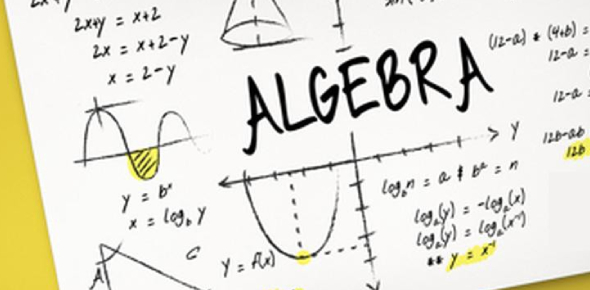10 Questions | Attempts: 9806
ShareSettingsHey, if you're looking for some new and unique algebra quiz questions and answers for grade 9th students, you've come to the right place. The below quiz is designed to test your understanding of the topics covered. Algebra is considered the most challenging subject by most people in grade nine, but this is essentially not true as all that one needs to understand to practice after covering every topic. Do you need some help with Algebra? Start by taking this quiz and watch out. Good luck!

• 1.
The sum of 2 numbers is 46. One number is 12 more than the other. What are the numbers?
• A.

12, 19

• B.

17, 29

• C.

32, 6

• D.

18, 30

• 2.
Evaluate this equation for the given value of a and b.  a2 + b2 , for a = 2 and b = 2
• A.

6

• B.

8

• C.

18

• D.

20

• 3.
The sum of 3 consecutive whole numbers is 108. What are the numbers?
• A.

34, 35, 36

• B.

33, 34, 35

• C.

35, 36, 37

• D.

31, 32, 33

• 4.
Jack is 5 years older than Mary. In four years the sum of their age is 33 years old. What are their ages?
• A.

Mary is 10 years old, Jack is 15 years old

• B.

Mary is 9 years old, Jack is 19 years old

• C.

Mary is 12 years old, Jack is 17 years old

• D.

Mary is 8 years old, Jack is 19 years old

• 5.
A basketball court is a rectangle with dimensions 29m by 16m. What is the length of a diagonalm to the nearest metre?
• A.

29

• B.

30

• C.

31

• D.

32

• 6.
Large pizzas cost \$12.50 and small pizzas cost \$9.00. The pizza parlor sold 38 pizzas with a total value of \$415.50. How many of each type did the pizza parlor sell?
• A.

20 large, 17 small

• B.

22 large, 18 small

• C.

21 large, 17 small

• D.

23 large, 18 small

• 7.
Find the value of x in this equation:  (x - 4) / (- 6) = 3
• A.

-14

• B.

-10

• C.

-24

• D.

14

• 8.
Simplify this equation:  (-5x - 10) / (x + 2)
• A.

5

• B.

-5

• C.

-15

• D.

10

• 9.
What are the x and y intercepts of the line 2x−3y+6=0?
• A.

3, 2

• B.

2, 3

• C.

3, -2

• D.

-3, 2

• 10.
What will be the slope of the line with equation y - 4 = 10?
• A.

5

• B.

4

• C.

8

• D.

0

## Related TopicsBack to top
×

Wait!
Here's an interesting quiz for you.